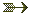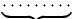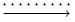# All commands, alphabetic order; letter U

## \u

The \u command generates a breve accent. The translation of

\u A \u a \u{\A} \u{\a} \u{\'A} \u{\'a} \u{\h A} \u{\h a} \u{\~A} \u{\~a}
\u{\d A} \u{\d a}\\ \u E \u e \u{\c E} \u{\c e} \u G \u g \u I \u i
\u O \u o \u U \u u


is the following list of characters Ă ă Ằ ằ Ắ ắ Ẳ ẳ Ẵ ẵ Ặ ặ Ĕ ĕ Ḝ ḝ Ğ ğ Ĭ ĭ Ŏ ŏ Ŭ ŭ

 &#258;  &#259; &#7856; &#7857; &#7854; &#7855; &#7858; &#7859; &#7860; &#7861;
&#7862; &#7863;  &#276;  &#277; &#7708; &#7709;  &#286;  &#287;  &#300;  &#301;
&#334;  &#335;  &#364;  &#365;


## \uccode

When you say \uccode13 12, you change the uppercase code of the character number 13 to the value 12. Both integers must be a valid character code. The \uppercase command replaces, in the token list given as argument, every character X by the character Y, provided that \uccode X is Y, and Y is not zero; all other tokens are left unchanged. See \uppercase.
A character code is an integer between 0 and N-1, where N=256 in TeX and in old versions of Tralics; it is now 65536. In Tralics, \lccode and \uccode are defined for all letters of iso-8859-1 (with, and without accents), these are characters with code less than 256 (for all other characters, \lccode and \uccode are zero).See scanint for details of how numbers are read.

## \uchyph (internal integer)

When you say \uchyph=78, (or any positive integer) then TeX accepts to hyphenate words starting with an uppercase letter. Tralics hyphenates nothing.(See scanint for details of argument scanning).

## \ul

Command from the soul package, that takes an argument and behaves like \textit. The command name \so stands for space-out, \caps for capitals, \hl is for highlight, \so is for strike-out, \st for stoke out, \ul is for underline. These commands take one argument, and translate it normally. Example

\caps{ABC}\hl{x\ul{y}\textit{z\st{w}}}\ul{etc}


Translation

<hi rend='caps'>ABC</hi>
<hi rend='hl'>x<hi rend='ul'>y</hi>
<hi rend='it'>z<hi rend='st'>w</hi></hi></hi>
<hi rend='ul'>etc</hi>


If the configuration file contains the following lines

  xml_caps_name="font-soul-caps"
xml_ul_name="font-soul-ul"
xml_hl_name="font-soul-hl"
xml_so_name="font-soul-so"
xml_st_name="font-soul-st"
xml_font_it = "font-italic-shape"
use_font_elt = "true"


then translation will be

<font-soul-caps>ABC</font-soul-caps>
<font-soul-hl>x<font-soul-ul>y</font-soul-ul>
<font-italic-shape>z<font-soul-st>w</font-soul-st></font-italic-shape>
</font-soul-hl>
<font-soul-ul>etc</font-soul-ul>


## \UndefineShortVerb

This command undefines a short hand character for verbatim code. The command takes one argument that is a one-character command, for instance \UndefineShortVerb{\+}. After that, $1+1$ works again.For more details, see the catcode section.

## \underaccent

The \underaccent command is valid only in math mode. It takes two arguments A and B, and puts A as an underaccent to B. For instance, the translation of \underaccent {\mathmo {^^^^02dc}}x is <munder accentunder='true'> <mi>x</mi> <mo>&#x2DC;</mo> </munder>. The funny character is a tilde accent. Other characters could be used. For instance \underbrace uses character U+FE38, \underleftarrow uses a leftarrow (U+2190), \underleftrightarrow uses a leftrightarrow (U+2194), \underrightarrow uses a rightarrow (U+2192). Note that \underset puts also A under B, but does not set the underaccent property.

## \underbrace

The \underbrace command puts an horizontal brace under a kernel. It works only in math mode. Example.See also the \acute command or \underaccent.

## \underleftrightarrow

The \underleftarrow command puts an arrow accent (pointing to the left) under a kernel. It works only in math mode. See also the \acute command or \underaccent.

## \underleftrightarrow

The \underleftrightarrow command puts an arrow accent (pointing to the left and the right) under a kernel. It works only in math mode. See also the \acute command or \underaccent.

## \underline

The \underline command puts a line under a kernel. It works in text mode as well as in math mode.See also the \acute command. See for an example in text mode \rm command.

## \underrightarrow

The \underrightarrow command puts an arrow accent (pointing to the right) under a kernel. It works only in math mode.See also the \acute command or \underaccent.

## \underset

The \underset command takes two arguments A and B, it places A (superscript size) under B. It works only in math mode.See also the \acute command. See also \underaccent.

## \undertilde

The \undertilde command puts a tilde character under a kernel. It works only in math mode; See \underaccent.

## \unexpanded (eTeX extension)

The command \unexpanded reads a token list, returns the list unchanged. This command behaves like \the, in that the resulting token list is not expanded, even in a \edef or \write. In the example here, the expansion of the first \foo is delayed. Command \bad is never executed.

\def\foo{12}\def\equals{<}
\edef\A{\unexpanded{\foo\equals}\foo\relax}
\def\equals{=}
\def\foo{11}


## \unhbox

The \unhbox command reads an integer N (a register code, see scanint for details), and adds the content of the box number N to the current list. After that, the box is empty. In Tralics, there is no difference between \unhbox and \unvbox, neither between \unhcopy and \unvcopy. There is a major difference between \unhcopy and \copy: in one case, we use a copy of the content, in the other case a copy of the box. Note: a register code is a small number between 0 and 255 in TeX, is much larger in ε-TeX, and is at most 1023 in current implementation of Tralics.

Example.

\def\makeboxes{%
\setbox0=\hbox{123}%
\setbox1=\xbox{foo}{456}%
\setbox2=\xbox{foo}{789\xbox{bar}{abc}}}
1.\makeboxes \copy0 \copy1 \copy2 \copy0 \copy1 \copy2\par
2.\makeboxes \box0 \box1 \box2 \box0 \box1 \box2\par
3.\makeboxes \unhcopy0 \unhcopy1 \unhcopy2 \unhcopy0 \unhcopy1 \unhcopy2\par
4.\makeboxes \unhbox0 \unhbox1 \unhbox2 \unhbox0 \unhbox1 \unhbox2\par
5.\makeboxes \unvcopy0 \unvcopy1 \unvcopy2 \unvcopy0 \unvcopy1 \unvcopy2\par
6.\makeboxes \unvbox0 \unvbox1 \unvbox2 \unvbox0 \unvbox1 \unvbox2\par


Translation.

<p>1.123<foo>456</foo><foo>789<bar>abc</bar></foo>123<foo>456</foo><foo>789<bar>abc</bar></foo></p>
<p>2.123<foo>456</foo><foo>789<bar>abc</bar></foo></p>
<p>3.123456789<bar>abc</bar>123456789<bar>abc</bar></p>
<p>4.123456789<bar>abc</bar></p>
<p>5.123456789<bar>abc</bar>123456789<bar>abc</bar></p>
<p>6.123456789<bar>abc</bar></p>


## \unhcopy

The \unhcopy command reads an integer N (a register code, see scanint for details), and returns a copy of the content of the box number N to the current list. Note: a register code is a small number between 0 and 255 in TeX, is much larger in ε-TeX, and is at most 1023 in current implementation of Tralics. See \unhbox for an example.

## \unitlength

This is a dimension, set initially to one pt. You modify it via \setlength\unitlength{2cm}. It is used by the picture environment and commands used in it,See picture environment. (this can be modified via \unitlength=2cm, see scandimen for details of argument scanning in this case).

## \unkern

The purpose of this command is to remove the last item on the current list, if it is a kern. Since Tralics does not maintain a list of items like TeX, this command does nothing.

## \unless (eTeX extension)

The \unless command is an extension of ε-TeX; the behavior of \unless\iftrue is the same as \iffalse. This means the following: This command is expandable; it reads a token; this token must be a conditional, but not \ifcase. The conditional computes a truth value, which is then negated. Expansion of the command is the same as that of the conditional, said otherwise, the next token, if the test is true, otherwise what follows the \else or \fi.

## \unlhd (math symbol)

The command \unlhd is valid in math mode only, its translation is <mo>&#x22B4;</mo>, ⊴.

## \unnumberedverbatim (Tralics command)

After the \unnumberedverbatim command is issued, lines produced by the verbatim are no more preceded by a line number.

## \unpenalty

The purpose of this command is to remove the last item on the current list, if it is a penalty. Since Tralics does not maintain a list of items like TeX, this command does nothing.

## \unpresetkeys, \unsavekeys

These commands are provided by the xkeyval package.

## \unrhd (math symbol)

The command \unrhd is valid in math mode only, its translation is <mo>&#x22B5;</mo>, ⊵.

## \unskip

The purpose of this command is to remove the last item on the current list, if it is a glue. In Tralics a single space is removed. The translation of a \space\unskip b \unskip c\space \unskip d\space\space \unskip\unskip e is a bcde.

## \unvbox

The \unvbox command reads an integer N (a register code, see scanint for details), and adds the content of the box number N to the current list. After that, the box is empty. See \unhbox for an example. Note: a register code is a small number between 0 and 255 in TeX, is much larger in ε-TeX, and is at most 1023 in current implementation of Tralics.

## \unvcopy

The \unvcopy command reads an integer N (a register code, see scanint for details), and returns a copy of the content of the box number N to the current list. Note: a register code is a small number between 0 and 255 in TeX, is much larger in ε-TeX, and is at most 1023 in current implementation of Tralics.See \unhbox for an example.

## \uparrow (math symbol)

The \uparrow command is valid only in math mode. It generates an arrow <mo>&uparrow;</mo> (Unicode U+2191, ↑). See description of the \leftarrow command.

## \Uparrow (math symbol)

The \Uparrow command is valid only in math mode. It generates an arrow: <mo>&Uparrow;</mo> (Unicode U+21D1, ⇑). See description of the \leftarrow command.

## \updownarrow (math symbol)

The \updownarrow command is valid only in math mode. It generates an arrow: <mo>&updownarrow;</mo> (Unicode U+2195, ↕). See description of the \leftarrow command.

## \Updownarrow (math symbol)

The \Updownarrow command is valid only in math mode. It generates an arrow: <mo>&Updownarrow;</mo> (Unicode U+21D5, ⇕). See description of the \leftarrow command.

## \upharpoonleft (math symbol)

The \upharpoonleft command is valid only in math mode. It generates a harpoon: <mo>&upharpoonleft;</mo> (Unicode U+21BF, ↿).

## \upharpoonright (math symbol)

The \upharpoonright command is valid only in math mode. It generates a harpoon: <mo>&upharpoonright;</mo> (Unicode U+21BE, ↾).

## \uplus (math symbol)

The \uplus command is valid only in math mode. It generates a binary operator: <mo>&uplus;</mo> (Unicode U+228E, ⊎). See description of the \pm command.

## \uppercase

The \uppercase command converts its argument to uppercase. (see scantoks for the syntax).

The \uppercase and \lowercase commands take as argument a general text, and convert the balanced text to uppercase form or lowercase form. The balanced text is then read again. Example: UPPER\uppercase \relax\space \bgroup }\lowercase \relax\space \bgroup }CASE. This is the same as UPPERCASE.

Here is an example (from the latex kernel).


\begingroup
\catcodeP=12
\catcodeT=12
\lowercase{
\def\x{\def\rem@pt##1.##2PT{##1\ifnum##2>\z@.##2\fi}}}
\expandafter\endgroup\x
\def\strip@pt{\expandafter\rem@pt\the}


What happens is the following. Inside a group, the catcodes (category codes) of the letters P and T are changed to 12, and \lowercase is called. All characters are converted from upper case to lower case. But the list contains only two such characters: P and T. It contains some commands like \def, \rem@pt, etc, that are unaffected, as well as the digits, the sharp sign and the dot. The effect of the \lowercase, the brace that follows, and the line that follows is as if you had said: \def\x {\def\rem@pt ##1.##2pt{##1\ifnum ##2>\z@ .##2\fi}} but where the second pt' is formed of characters of catcode 12 (note: if you try \catcodet=12 \catcode\p=12 \def\x{...} you are in trouble because TeX sees \ca followed by a t. If you swap the assignments, you cannot create the token \rem@pt).

The effect of the \expandafter is to push the following token list \def\rem@pt#1.#2pt{#1\ifnum#2>\z@.#2\fi} after the \endgroup, thus to define \rem@pt outside the group in which the catcodes have changed. This command cannot be used as \rem@pt1.2pt, because in such a construct, both p have the same catcode. However, the tokens that result from the expansion of \the have the desired catcodes. Hence \stri@pt can be put before a dimension. Example.

\dimen0=3pt
\dimen1=3.4pt
\strip@pt\dimen0
\strip@pt\dimen1
\kern\strip@pt\fontdimen1\font\dimen@


This is the same as 33.4 followed by a kern whose value is the slant of the current fontimes some dimension.

There are two tables that control conversion: \lccode 1=2 says that \lowercase should convert character 1 into character 2, and \uccode 3=4 says that \uppercase should convert character 3 into character 4.

The example that follows is from the LaTeX kernel, it defines a command that takes an argument, say A or \A. The macro uses #1 in a context where an integer is required; so that in both cases the number 65 is used. Notice that one of these #1 is inside the scope of \lowercase, so that using A as argument is not a good idea, because sometimes it will produce a lower case a. The effect of the macro is to make the character A active and to define it be \char65 (this is letter A), preceded by a kern of width zero (preventing ligatures with a preceding character) preceded by \leavevmode (for the case where a paragraph has to be started). The interesting point is how to define the character. The magic is \lowercase{\endgroup\def~{...}}. This is the same as \endgroup\def A{...}, where all characters have been replaced by their lower case equivalent; for instance the ~ is replaced by A. The important point is that the category code of the characters do not change: the active tilde is replaced by an active A. Note that the \def is in the scope of the \lowercase, but out of the group in which the the category code of the tilde has changed.

\def\do@noligs#1{%
\catcode#1\active
\begingroup
\lccode\~#1\relax
\lowercase{\endgroup\def~{\leavevmode\kern\z@\char#1}}}


This is another example. This is a loop, for each number from 0 to 127. In the loop, the null character (entered as ^^@) is active, and its uppercase equivalent takes all ASCII values.

There are two definitions. Assume that \count@ contains 65. The first \edef defines is an active A, the second defines \8:A. Note that \string^^@ is a letter A, of catcode 12, \noexpand^^@ is an active A (not-expanded) and \catcode\count@ is the catcode of the letter A.

Example (from xmltex.tex, for utf-8 parsing).

\endlinechar-1
\count@0
\catcode0=13
\gdef\XML@tempa{
\begingroup
\uccode0\count@
\uppercase{\endgroup
\edef^^@{
\ifnum\catcode\count@=11 %
\noexpand\utfeightay\else\noexpand\utfeightax\fi
\noexpand^^@}
\expandafter\edef\csname 8:\string^^@\endcsname{\string^^@}}
\XML@tempa


## \upshape

The \upshape command changes the shape of the current font to a upright shape. For an example of fonts, see \rm.

## \upsilon (math symbol)

The \upsilon command is valid only in math mode. It generates a Greek letter: υ <mi>&upsilon;</mi> (Unicode U+3C5, υ).

## \Upsilon (mathsymbol)

The \Upsilon command is valid only in math mode. It generates an uppercase Greek letter: Υ <mi>&Upsilon;</mi> (Unicode U+3A5, Υ).

## \upuparrows (math symbol)

The \upuparrows command is valid only in math mode. It generates <mo>&upuparrows;</mo> a double upwards arrow, Unicode character U+21C8, ⇈.

## \url

The \url command takes one argument X. The translation is a <xref> whose content is X, and that has a url attribute whose value is also X. In the case of \href, the second argument is traditionally a \url command; in this case the \url command just translates the argument. Inside the \url command, underscore and tilde characters are considered normal characters. Moreover, \~ is handled in the same fashion as a tilde. Since urls can be long, Tralics adds a special marker <allowbreak> wherever it is wise to split the url, namely after a slash or a dot. No such markers are added when the starred version \url* is used. For instance, the translation of

\href{a~b\~n_d}{\url{a~b\~n_d}}
\url{a~b\~n_d}
\url{http://www.inria.fr}
\url*{http://www.inria.fr}


is

<xref url='a~b~n_d'>a~b~n_d</xref>
<xref url='a~b~n_d'>a~b~n_d</xref>
<xref url='http://www.inria.fr'>http://<allowbreak/>www.<allowbreak/>inria.<allowbreak/>fr</xref>
<xref url='http://www.inria.fr'>http://www.inria.fr</xref>
`

## \urlfont

The \urlfont command explains what font to use for URLs. See \href. This command is specific to Tralics.

## \usebox

You say \usebox{17} or \usebox{\foo}, where \foo evaluates to a box number; this inserts a copy of the box into the XML file, in the same way as \leavevmode\copy 17\relax.

## \usefont

In LaTeX, you can say \usefont{OML}{\rmdefault}{\f@series}{it} if you want to select old style numerals. In Tralics, things are a bit more complicated; first, the two commands in the arguments 2 and 3 are not necessarily defined. The command takes four arguments, an encoding, a family, a series and a shape. The first argument is ignored by Tralics. Others are used to select a font.For an example see \fontfamily.

## \usepackage

The \usepackage command can be used only before the \begin{document}. It takes one argument, say pk preceded by an optional argument (say opts), followed by an optional argument (say date). In case pk is calc or fp then command from the calc or fp packages are available. If it is french or frenchle then the default language is French. It it is babel, then the default language is set the French or English, depending on whether french (with variants francais and frenchb) or english is present in opt.

If the argument is foo, then the style file foo.plt is read, in a special manner. The file is read only once, even if the command is issued more than once (but the optional arguments should be the same). No error is signaled if the file is not found. The @ character is a normal character while reading the style file. The style file has an identification line that defines a date, that should not be older than the date parameter (use the format 1999/12/31 for the last day of 1999). The opts is a comma separated list of options, the file defines an action for each option. The order of these options is sometimes relevant, they are parsed from left to right.

## \UseVerb

There are cases where verbatim material is forbidden. The solution consists of reading the verbatim material and putting it in a command, then use it later. The \SaveVerb command takes as argument a name (a sequence of letters), and reads the verbatim material. This material is saved, and can be used by the \UseVerb command. For an example, see catcode section.back to home page © INRIA 2003-2005, 2006, 2008 Last modified $Date: 2015/08/04 15:54:02$